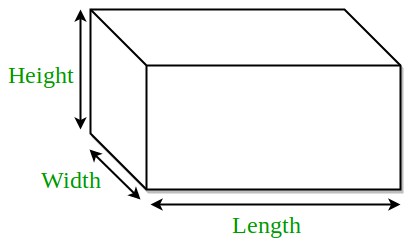# Program for Volume and Surface Area of Cuboid

Cuboid is a 3-dimensional box-like figure represented in the 3-dimensional plane.Cuboid has 6 rectangled-shape faces. Each face meet another face at 90 degree each.Three sides of cuboid meet at same vertex.Since it is made up of 6 rectangle faces, it have length, width and height of different dimension.Examples :

```Input : 2 3 4
Output : Area = 24
Total Surface Area = 52

Input : 5 6 12
Output : Area = 360
Total Surface Area = 324```

Formulae :

```Area = l*w*h
Total Surface Area = 2*l*w + 2*w*h + 2*l*h
where l, h, w are length, height and width of
cuboid respectively.```

## C++

 `// CPP program to find volume and ` `// total surface area of cuboid ` `#include ` `using` `namespace` `std; ` ` `  `// utility function ` `double` `areaCuboid(``double` `l, ``double` `h, ``double` `w) ` `{ ` `    ``return` `(l * h * w); ` `} ` ` `  `double` `surfaceAreaCuboid(``double` `l, ``double` `h, ``double` `w) ` `{ ` `    ``return` `(2 * l * w + 2 * w * h + 2 * l * h); ` `} ` ` `  `// driver function ` `int` `main() ` `{ ` `    ``double` `l = 1; ` `    ``double` `h = 5; ` `    ``double` `w = 7; ` `    ``cout << ``"Area = "` `<< areaCuboid(l, h, w) << endl; ` `    ``cout << ``"Total Surface Area = "`  `         ``<< surfaceAreaCuboid(l, h, w); ` `    ``return` `0; ` `} `

## Java

 `// Java program to find volume and ` `// total surface area of cuboid ` ` `  `class` `GFG  ` `{ ` `    ``// utility function ` `    ``static` `double` `areaCuboid(``double` `l, ``double` `h,  ` `                                           ``double` `w) ` `    ``{ ` `        ``return` `(l * h * w); ` `    ``} ` ` `  `    ``static` `double` `surfaceAreaCuboid(``double` `l, ``double` `h,  ` `                                                ``double` `w) ` `    ``{ ` `        ``return` `(``2` `* l * w + ``2` `* w * h + ``2` `* l * h); ` `    ``} ` `     `  `    ``// Driver code ` `    ``public` `static` `void` `main (String[] args)  ` `    ``{ ` `        ``double` `l = ``1``; ` `        ``double` `h = ``5``; ` `        ``double` `w = ``7``; ` `        ``System.out.println(``"Area = "` `+ areaCuboid(l, h, w)); ` `        ``System.out.println(``"Total Surface Area = "` `                            ``+ surfaceAreaCuboid(l, h, w)); ` `    ``} ` `} ` `// This code is contributed By Anant Agarwal. `

## Python3

 `# Python3 code to find volume and ` `# total surface area of cuboid ` ` `  `# utility function ` `def` `volumeCuboid( l , h , w ): ` `    ``return` `(l ``*` `h ``*` `w) ` `     `  `def` `surfaceAreaCuboid( l , h , w ): ` `    ``return` `(``2` `*` `l ``*` `w ``+` `2` `*` `w ``*` `h ``+` `2` `*` `l ``*` `h) ` ` `  `# driver function ` `l ``=` `1` `h ``=` `5` `w ``=` `7` `print``(``"Volume ="` `, volumeCuboid(l, h, w)) ` `print``(``"Total Surface Area ="``, surfaceAreaCuboid(l, h, w)) ` ` `  `#This code is contributed by "Sharad_Bhardwaj". `

## C#

 `// C# program to find volume and ` `// total surface area of cuboid ` `using` `System; ` ` `  `class` `GFG { ` `     `  `    ``// utility function ` `    ``static` `double` `areaCuboid(``double` `l, ``double` `h, ` `                            ``double` `w) ` `    ``{ ` `        ``return` `(l * h * w); ` `    ``} ` ` `  `    ``static` `double` `surfaceAreaCuboid(``double` `l, ``double` `h, ` `                                    ``double` `w) ` `    ``{ ` `        ``return` `(2 * l * w + 2 * w * h + 2 * l * h); ` `    ``} ` ` `  `    ``// Driver code ` `    ``public` `static` `void` `Main() ` `    ``{ ` `        ``double` `l = 1; ` `        ``double` `h = 5; ` `        ``double` `w = 7; ` `         `  `        ``Console.WriteLine(``"Area = "` `+ areaCuboid(l, h, w)); ` `        ``Console.WriteLine(``"Total Surface Area = "` `                        ``+ surfaceAreaCuboid(l, h, w)); ` `    ``} ` `} ` ` `  `// This code is contributed By vt_m. `

## PHP

 ` `

## Javascript

 ``

Output :

```Area = 35
Total Surface Area = 94```

Time complexity : O(1)
Auxiliary Space : O(1)

If you like GeeksforGeeks and would like to contribute, you can also write an article using write.geeksforgeeks.org or mail your article to review-team@geeksforgeeks.org. See your article appearing on the GeeksforGeeks main page and help other Geeks.